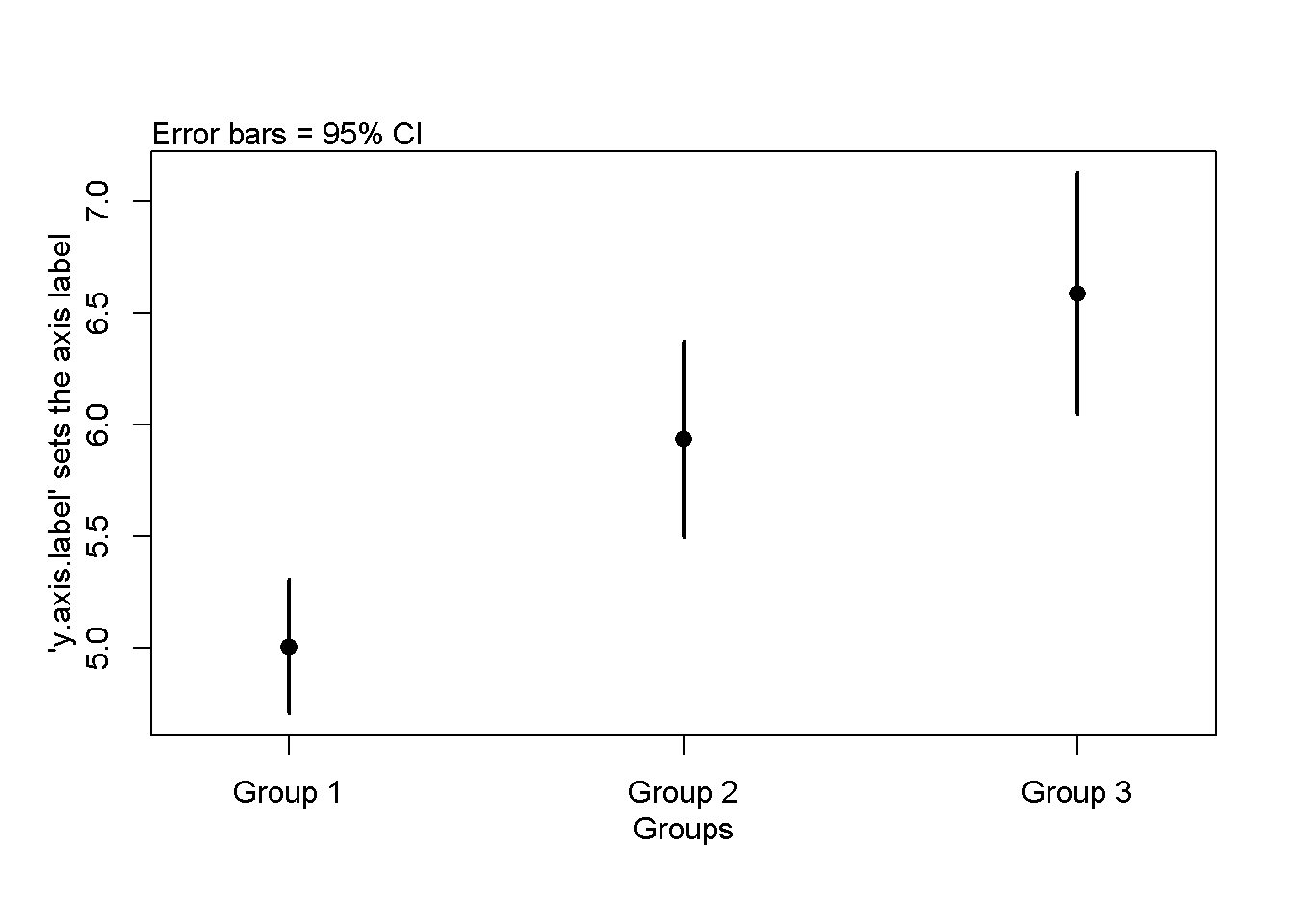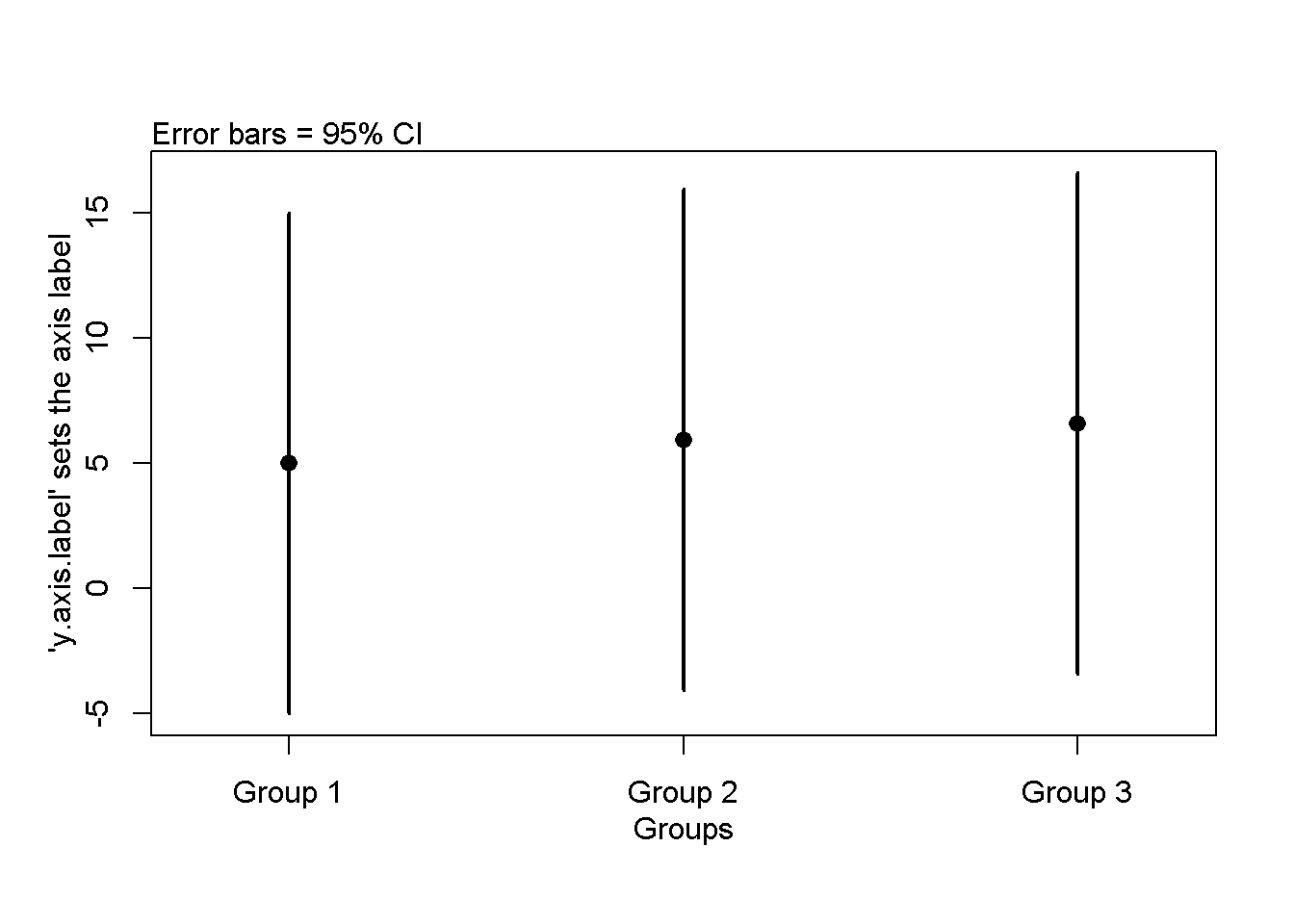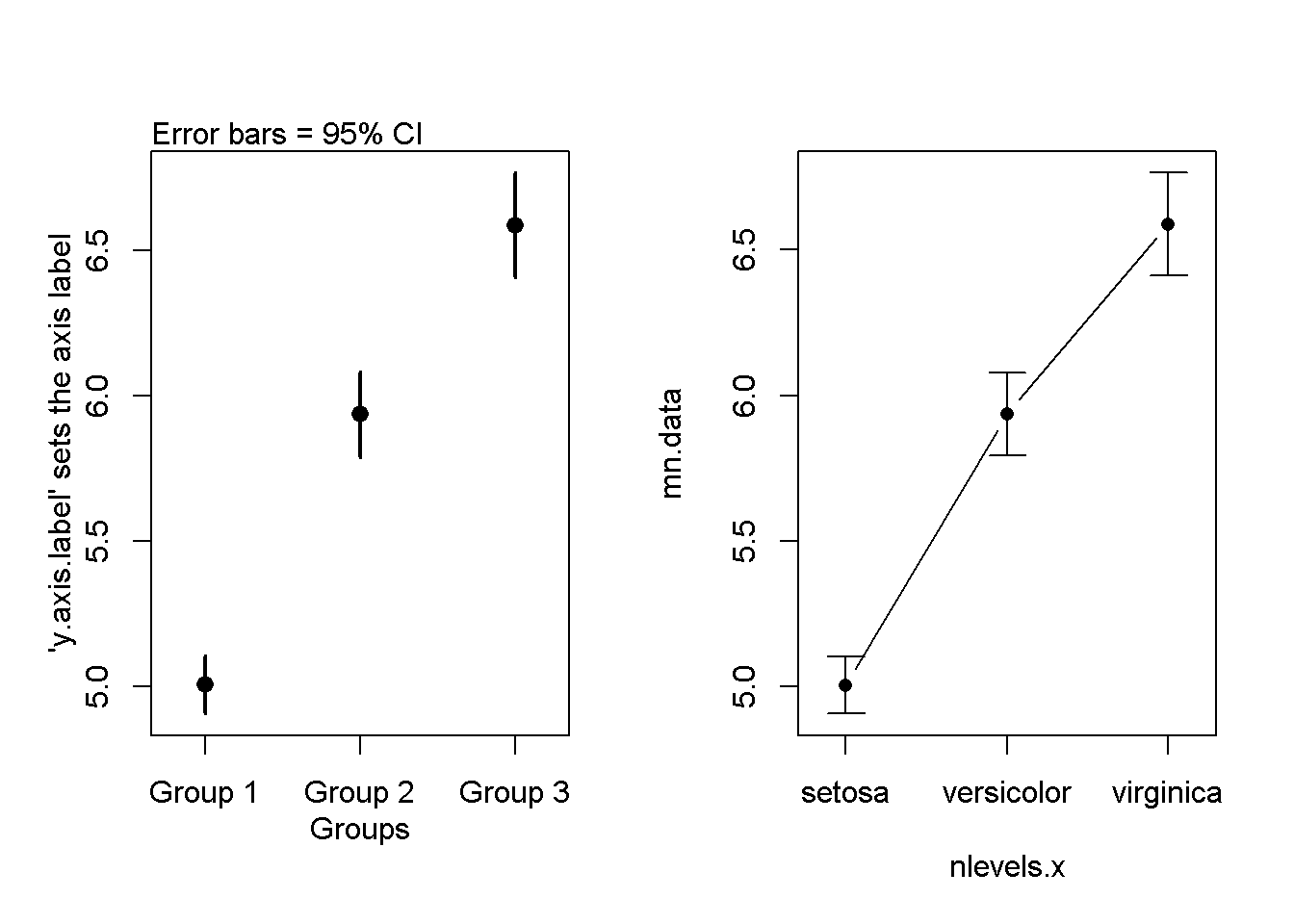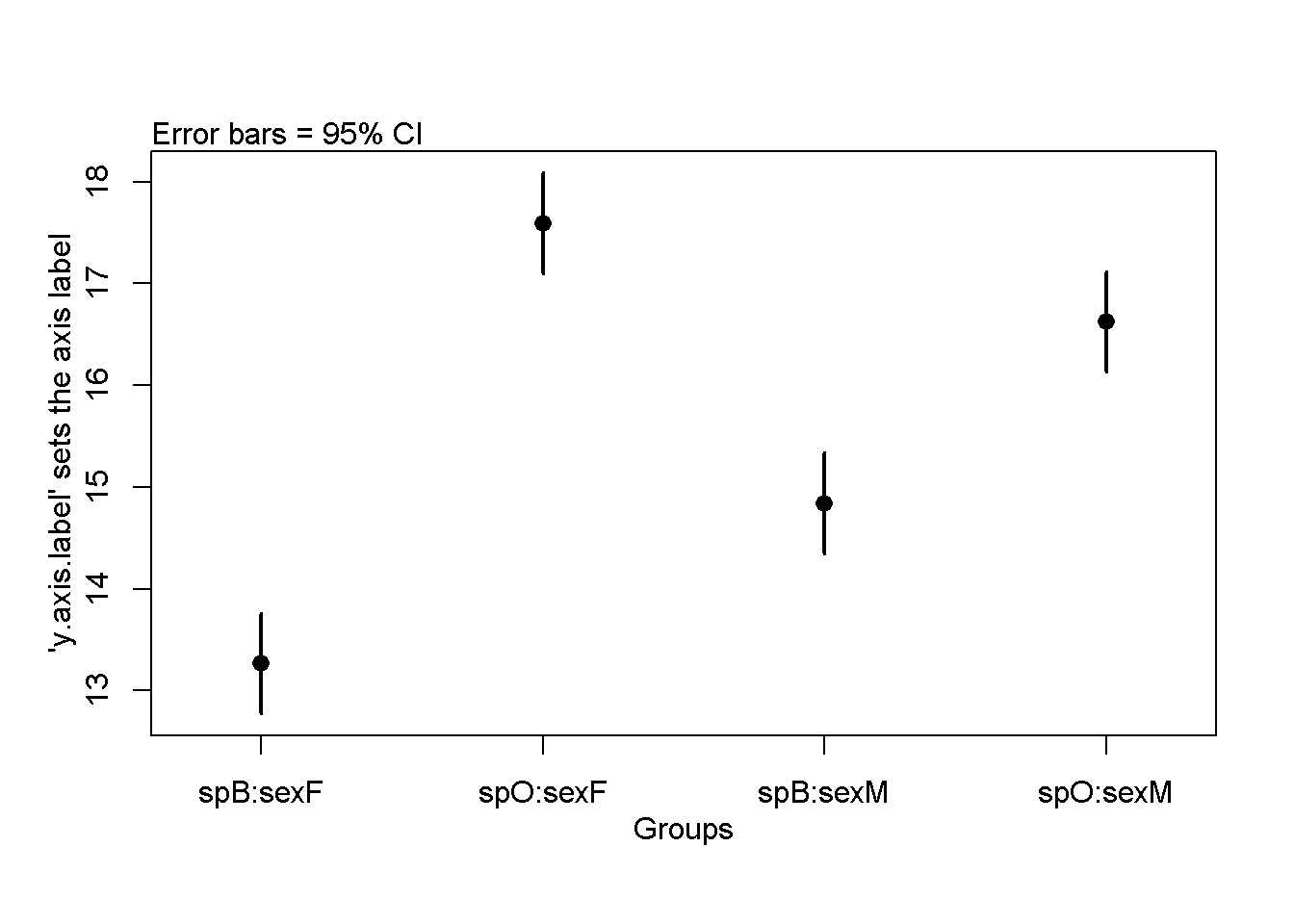Changes to this code will be announced via email and the course FaceBook page CalU EcoStats.

For more labs and tutorials see my WordPress Site lo.brow.R

Intro

This is a simple function that plots means as individual points (not bars) and approximate 95% confidence intervals using user-supplied means and standard errors or 95% CIs. It provides some feed back via the console and the plot itself regarding errors and how to make the plot more polished.

Base R has no default function to make plots like this, though there are numerous packages that have function that can do this (sciplot, ggplot, Hmisc, gplot, psych). I wrote this function as bare-bones alternative that my students could load via a script and which has only a few, simple arguements. I teach them to calculate means and standard deviations using the tapply() function.

I’ve found that loading packages can be difficult on computers behind campus firewalls, as can updating R if a package requires an up-to-date installation. At the end of this document I provide notes about and links to other ways to approach this problem with R published packages.

plot.means()

# means = your means. , contained in a vector, eg c(mean1, means2...)
# SEs =  your standard errors, contained in a vector,
# CI.hi = upper confidence intervals, in a vector,
# CI.lo = lower confidence itnerval, in a vector
# categories = the names of the categories/groups, in the order that they appear
# x.axis.label = what should be plotted on the x axis
# y.axis.label = what should be plotted on the y axis
#### The function STARTS here ####
plot.means <- function(means = NULL,
SEs = NULL,
CI.hi = NULL,
CI.lo = NULL,
categories = NULL,
x.axis.label = "Groups",
y.axis.label = "'y.axis.label' sets the axis label",

# Error messages
if(is.null(means)==T){
stop("No means entered") }

if(is.null(SEs)==T & is.null(CI.lo) == T){
stop("No standard errors entered") }

if(is.null(CI.lo) != T &  is.null(CI.hi)){
stop("CI.lo entered but no CI.hi") }

if(is.null(CI.hi) != T &  is.null(CI.lo)){
stop("CI.hi entered but no CI.lo") }

#check if both SE and and CI.is enter
if(is.null(SEs) == F &  is.null(CI.hi) == F){
stop("Both SEs and CI.hi entered; use eithe SEs or CIs") }

if(is.null(SEs) == F &  is.null(CI.lo) == F){
stop("Both SEs and CI.lo entered; use eithe SEs or CIs") }

#Check if the number of means matches the number of SE
n.means <- length(means)
n.SEs    <- length(SEs)
n.CI.lo  <- length(CI.lo)
n.CI.hi  <- length(CI.hi)

#CHeck standard errors against means
if(n.means != n.SEs & is.null(CI.lo)==T){
error.message <- paste("The number of means is",n.means,"but the number of standard errors is",n.SEs,sep = " ")
stop(error.message) }

#Check CIs against CIs
if(n.CI.lo != n.CI.hi){
error.message <- paste("The number of CI.lo is",n.CI.lo,"but the number of standard errors is",n.CI.hi,sep = " ")
stop(error.message) }

#assign arbitrary categories
if(is.null(categories) == T) {
categories <- paste("Group",1:n.means)
print("Set categoris labls with 'categories=' ")
}

# calculate values for plotting limits
if(is.null(SEs)==F){
}

# calculate values for plotting limits
if(is.null(SEs)==T){
}

#determine where to plot points along x-axis
x.values <- 1:n.means

#set x axis min/max
x.axis.min <- min(x.values)-0.05
x.axis.max <- max(x.values)+0.05

x.limits <- c(x.axis.min,x.axis.max)

#Plot means
plot(means ~ x.values,
xlim = x.limits,
ylim = c(y.min,y.max),
xaxt = "n",
xlab = "",
ylab = "",
cex = 1.25,
pch = 16)

axis(side = 1,
at = x.values,
labels = categories
)

# Plot confidence intervals
if(is.null(CI.hi) == FALSE &
is.null(CI.lo) == FALSE){
#Plot upper error bar for CIs
lwd. <- 2
arrows(y0 = means,
x0 = x.values,
y1 = CI.hi,
x1 = x.values,
length = 0,
lwd = lwd.)

#Plot lower error bar
arrows(y0 = means,
x0 = x.values,
y1 = CI.lo,
x1 = x.values,
length = 0,
lwd = lwd.)
}

# Estimate CIs from SEs

if(is.null(SEs) == FALSE &
is.null(CI.lo) == TRUE){
lwd. <- 2
arrows(y0 = means,
x0 = x.values,
y1 = means+2*SEs,
x1 = x.values,
length = 0,
lwd = lwd.)

#Plot lower error bar
arrows(y0 = means,
x0 = x.values,
y1 = means-2*SEs,
x1 = x.values,
length = 0,
lwd = lwd.)
}

mtext(text = x.axis.label,side = 1,line = 2)
mtext(text = y.axis.label,side = 2,line = 2)
mtext(text = "Error bars = 95% CI",side = 3,line = 0,adj = 0)

}

#### The function ENDS here ####
#### The function ENDS here ####
#### The function ENDS here ####
#### The function ENDS here ####
#### The function ENDS here ####

Test the function with 3 groups

The following code is used to test the function. DO NOT LOAD THIS CODE for general use

Calcualte mean and SE from Fisher’s iris data

# THIS IS NOT PART OF THE FUNCTION

data(iris)

iris.3mean <- tapply(iris$Sepal.Length, iris$Species,
mean)

iris.3sd <- tapply(iris$Sepal.Length, iris$Species,
sd)

iris.3n <- tapply(iris$Sepal.Length, iris$Species,
length)

iris.3se <- iris.3sd/sqrt(iris.3n)

Plot Fisher’s iris data with plot.means()

par(mfrow = c(1,1))
plot.means(means = iris.3mean,
SEs = iris.3se*3)
##  "Set categoris labls with 'categories=' "Test confidence interval function

Make fake confidence intervals

CI.hi <-iris.3mean+10
CI.lo <-iris.3mean-10

plot.means(means = iris.3mean,
CI.lo = CI.lo,
CI.hi = CI.hi)
##  "Set categoris labls with 'categories=' "Compare to the output of the sciplot package

This requires the sciplot package to be downloded

library(sciplot)
ci.fun.use <- function(x) c(mean(x)-1.96*se(x), mean(x)+1.96*se(x))
par(mfrow = c(1,2))
plot.means(means = iris.3mean,
SEs = iris.3se)
##  "Set categoris labls with 'categories=' "
lineplot.CI(x.factor = Species,
response = Sepal.Length,
ci.fun = ci.fun.use,
data = iris)Test the function with 4 groups

library(MASS)
data(crabs)
crab.means <- coef(lm(FL ~ -1 + sp:sex, data = crabs))
crab.categories <- names(crab.means)
crab.se.fake <- rep(sd(crabs\$FL)/sqrt(dim(crabs)),4)

par(mfrow = c(1,1))
plot.means(means = crab.means,
SEs = crab.se.fake,
categories = crab.categories)Other approaches

These approach vary in if/how they generate the intial plot of the means before adding the error bars.

Using ggplot w/geom_errorbar ggplot is the standard now for advanced graphics. http://www.cookbook-r.com/Graphs/Plotting_means_and_error_bars_(ggplot2)/

https://www.r-bloggers.com/line-plot-for-two-way-designs-using-ggplot2/

Using sciplot:lineplot.CI See examples above

Using psych::error.bars https://www.personality-project.org/r/html/error.bars.html

If you’re into “dynamite plots” https://www.r-bloggers.com/building-barplots-with-error-bars/ http://monkeysuncle.stanford.edu/?p=485